Courses

# Conservation of Energy and Momentum (Part - 2) Electrical Engineering (EE) Notes | EduRev

## Electrical Engineering (EE) : Conservation of Energy and Momentum (Part - 2) Electrical Engineering (EE) Notes | EduRev

The document Conservation of Energy and Momentum (Part - 2) Electrical Engineering (EE) Notes | EduRev is a part of the Electrical Engineering (EE) Course Electromagnetic Theory.
All you need of Electrical Engineering (EE) at this link: Electrical Engineering (EE)

In the last lecture, we had written down the expression for the rate of change of momentum of the sources and fields as given by,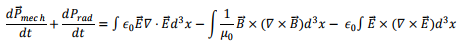We could express this equation in a symmetric fashion by adding a term prooportional to the divergence of the magnetic field (which is identically zero) and rewrite the above as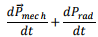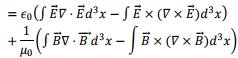We considered the pairs of term in parenthesis on the right and simplified their components as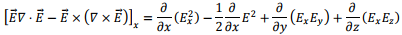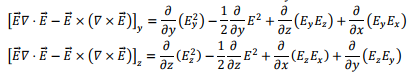Adding the second term of each of the components, we wrote it as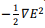and a similar contribution from the magnetic field gives us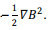Inserting the associated constants ∈0 and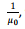we get this contribution as the gradient of energy density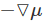which is the momentum density term.

We will now try to write the remaining terms in a compact fashion. We will simplify only the electric field terms and write down the corresponding magnetic field term by analogy. The remaining term are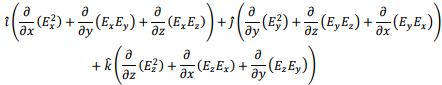Since the left hand side is a force, we would have liked to express it as a gradient of a scalar which would act like a potential. However, looking at the terms does not seem to suggest such a possibility because the components seem to have been mixed.

We , therefore, bring back the terms which we have been able to express as a gradient of the energy density and see whether there can be alternative ways of expressing them.

We note here that in expressing it as a gradient of a scalar, we started with a single function and obtained three quantities, the components of a vector. we are aware that if we start with a vector and take a divergence, we would get a scalar. Thus in some sense, the gradient increases the space but a divergence decreases the same. We generalize the definition of a vector to a “Tensor” of arbitrary rank n as a collection of 3n quantities. Thus a scalar is a tensor of rank zero, a vector a tensor of rank 1. In this case, we take a tensor of rank 2, denoted by nine components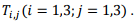A divergence of a tensor of rank 2 will reduce its rank by 1, giving us a vector which has 3 components.

We will first state the result and then show that this can be actually done. We claim that the right hand side of our force equation can be expressed as a divergence of a tensor of rank 2 denoted by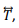whose components are given by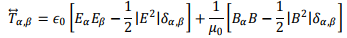To fix our ideas, let us once again, take B=0. We can expressas a 3 x 3 matrix as follows: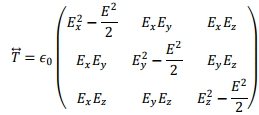Since the divergence of the tensor is a vector, it has three components and we define the components as follows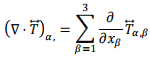Substituting the components given in the matrix above, we find,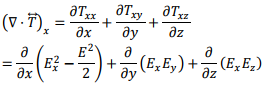which is precisely the x component of the electric field term that we had found. Similar statement can be proved for the y and z components and also for the magnetic field terms. Thus we have,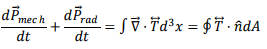where, we have written da for the area element instead of dA so as not to confuse with the Poynting vector which we have denoted by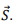The last relation is obtained by a procedure similar to the divergence theorem.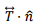is the momentum flux nornmal to the bounding surface of the volume. Since the relation is true for arbitrary volume, the relation can be stated in a differential form in terms of the momentum densities, indicated by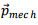and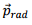as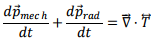This is a statement of the conservation of linear momentum for the electromagnetic field which sates that the rate of change of momentum of a closed system containing sources and fields can occur only through a transport of momentum through the bounding surface.

Examples

Force on one of the plates of a parallel plate capacitor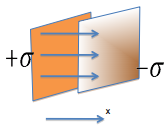Consider a parallel plate capacitor with charge densities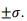Let the plates be in the yz plane so that the electric field is directed along the x-direction. we have,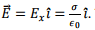Thus the Stress tensor in this case is diaginal and can be written as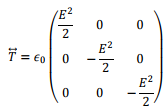The force acting on the negative plate is (assuming it is to the right of the positive plate) is along the negative x direction as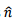is directed along – x direction. We have,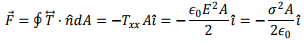Force on the Northern Hemisphere of a spinning charged sphere by the Souther Hemisphere

Though solving this problem can be done by more straightforward method, we are doing it by the method of Stress Tensor as an illustration.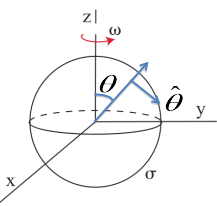First, let us consider the spinning shell. As the angular velocity is constant, the spinning shell is equivalent to a magnetic moment directed along the direction of the angular velocity vector. Let us take the angular velocity vector along the z direction.

The moving charges on the surface are equivalent to circulating current in the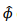direction. The surface current density is given by, in spherical polar,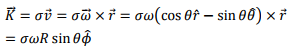where r=R  on the surface of the sphere. Consider a thin circular strip lying between angles θ and  θ + d θ.The radius of the circle is R sin θ.The width of the strip (which is crossed by the linear current density) is Rd θ so that the current flowing in the strip is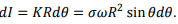The circulating loop is equivalent to a magnetic moment (πRsin2θ)dl in the z- direction. Since all such loops are parallel, the net magnetic moment of the spinning shell is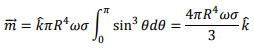The magnetic field due to the shell is given by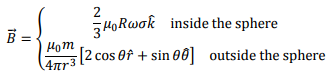Since the fields are constant, so is the Poynting vector. Thus the force is given by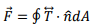By symmetry, the force is in z direction. Thus we will need to calculate only the z component of the normal component of the stress tensor. In this case, we consider only the magnetic forces (the electric force is also there as the shell has charges, but we restrict ourselves to magnetic case only. Since we are interested only in the z components, the only components of the stress tensor that we requires are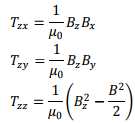In terms of these, we can write down the force as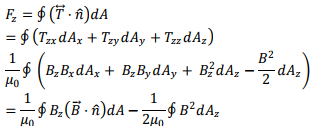As we are interested in calculating force on the northern hemisphere, there are two surfaces to take into account. The equatorial plane inside the sphere for which the normal direction is along the negative z direction. Since we need to consider only the inside field, the field to be considered here is constant.

The field is given by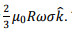Thus only term in stress tensor is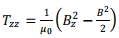and as the field is constant, the force is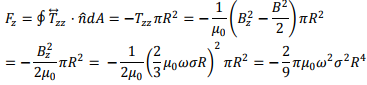That leaves us with the outside hemisphere. The normal to the surface is along the radial direction. We need to compute the integral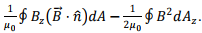Let us compute each term of the integrand using the expression for the field outside the shell, which is given by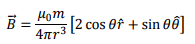Since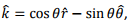we have,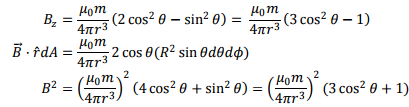Using these,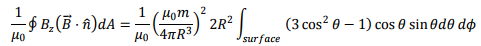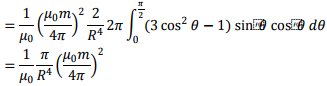the limits of integration are from 0 to π/2 as it is a hemisphere.

The second integral is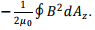and is given by, using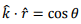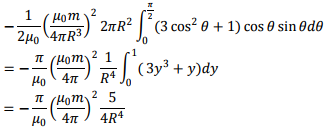Adding the two contributions, the force on the outer surface is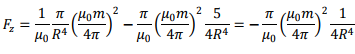Substituting the value of the magnetic moment,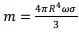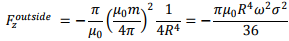If we add both the inside and the outside contributions, the magnetic force on the northern hemisphere is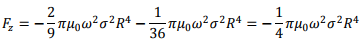the negative sign shows that the force is directed towards the southern hemisphere and is attractive in nature.

Offer running on EduRev: Apply code STAYHOME200 to get INR 200 off on our premium plan EduRev Infinity!

,

,

,

,

,

,

,

,

,

,

,

,

,

,

,

,

,

,

,

,

,

;